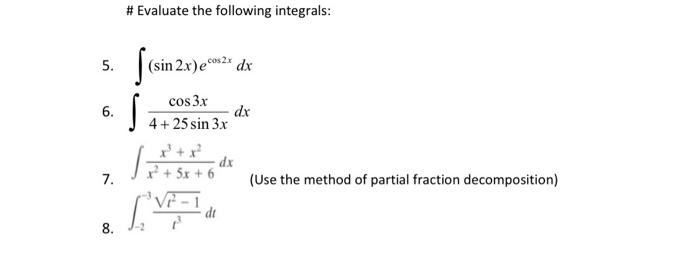### Create an Account

Home / Questions / #Evaluate the following integrals: 5. (sin 2.x)ece dx 6. cos 3x dx 4+25 sin 3x S Toiminta ...

# #Evaluate the following integrals: 5. (sin 2.x)ece dx 6. cos 3x dx 4+25 sin 3x S Toiminta dx 7. (Use the method of partial fraction decomposition) di 8.

#Evaluate the following integrals: 5. (sin 2.x)ece dx 6. cos 3x dx 4+25 sin 3x S Toiminta dx 7. (Use the method of partial fraction decomposition) di 8.

Apr 21 2021 View more View LessSubscribe To Get Solution